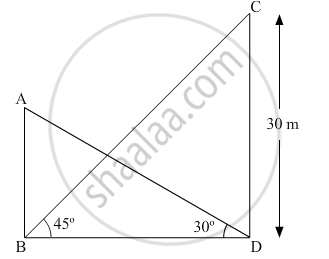# The angle of elevation of the top of a building from the foot of the tower is 30° and the angle of elevation of the top of the tower from the foot of the building is 45°. If the tower is 30 m high, find the height of the building. - Mathematics

The angle of elevation of the top of a building from the foot of the tower is 30° and the angle of elevation of the top of the tower from the foot of the building is 45°. If the tower is 30 m high, find the height of the building.

#### Solution

Let AB be the building and CD be the tower.In ΔCDB,

(CD)/(BD)=tan45^@

=>30/(BD)=1

BD=30 m

In ΔABD,

(AB)/(BD)=tan30^@

AB=BD × 1/sqrt3

=>AB=30/sqrt3=10sqrt3m

Therefore, the height of the building is 10sqrt3m

Concept: Heights and Distances
Is there an error in this question or solution?